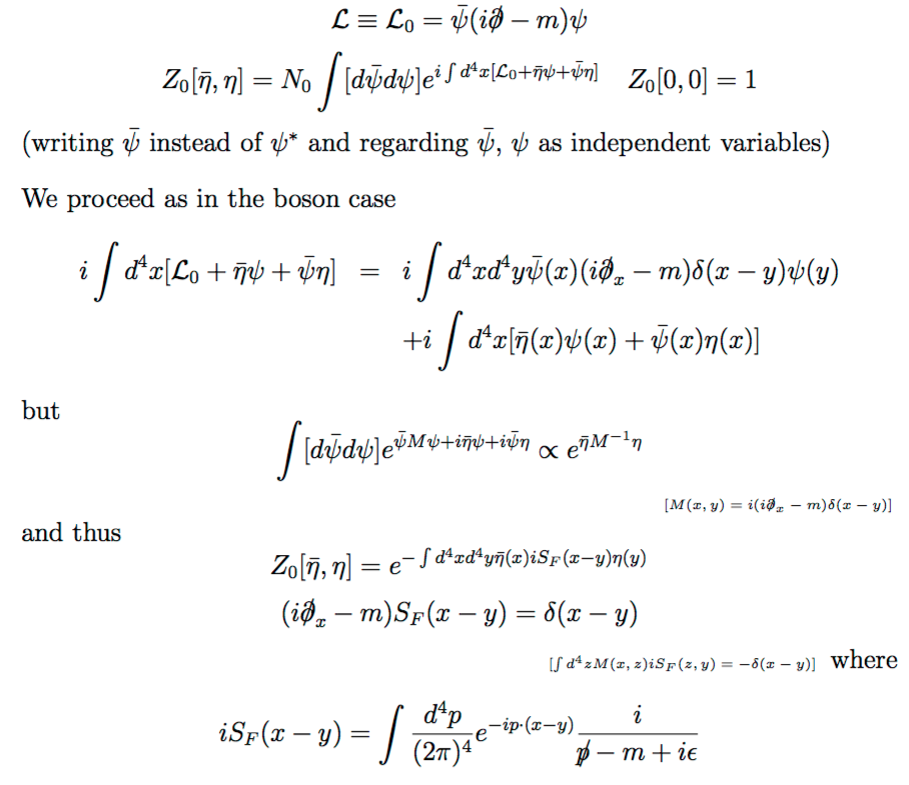# Generating Functional for the Dirac Field, equivalence of expressions

As with the Klein-Gordon field, we can alternatively derive the Feynman rules with the free Dirac theory by means of a generating functional. In analogy with the scalar field theory where $Z[J]$ is defined as$$Z[J] \equiv \mathcal{D}\phi\,\text{exp}\left[ i \int d^4x\left[\mathcal{L} + J(x)\phi(x)\right]\right],$$we define the Dirac generating functional as$$\require{slashed} Z[\overline{\eta}, \eta] = \int \mathcal{D} \overline{\psi}\,\mathcal{D}\psi\,\text{exp}\left[i \int d^4x[\overline{\psi}(i \partial\kern-0.5em\raise0.22ex\hbox{/} - m)\psi + \overline{\eta}\psi + \overline{\psi}\eta]\right],$$where $\eta(x)$ is a Grassmann-valued source field. It is not too hard to see that$$Z[\overline{\eta}, \eta] = Z_0 \cdot \text{exp}\left[ - \int d^4 x \,d^4y\,\overline{\eta}(x)S_F(x - y)\eta(y)\right].$$

To me, this is not so clear. Why does this follow?

• Which part is not clear? The part where you add the $\bar{\eta}\psi + \bar{\psi}\eta$ source term instead of $J\phi$? Or the part where a gaussian path integral gives you a gaussian generating function (which would be the same in the scalar case)? Nov 9, 2015 at 7:52